# Elementary Indices

Economics, Economic / Financial Data

Price indices such as the consumer price index (CPI) are usually calculated in two or more stages.  The later stages of the calculation involve using weighted methods described in the previous feature: Different ways of measuring the Consumer Price Index (CPI).  At the earliest stages of the calculation, elementary indices are used.

For a two stage calculation, the price figures used in the formulae described in the previous feature, are also price indices, and are usually calculated using the formulae described in this feature.  Elementary indices only apply to a single type of good.

An overall CPI might rely on several different types of elementary indices e.g. some good prices are estimated using the Dutot index, while Jevons index is applied to others.

For each of the following indices:
n is the sample size of the good being surveyed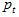are the prices of the good at the current period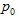are the prices of the good at the base period

## Dutot Index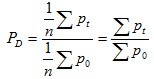## Jevons Index

Jevons index is the geometric mean of price ratios.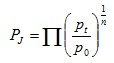## Carli Index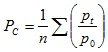## Harmonic Mean of Price Relatives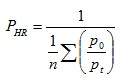## Carruthers / Sellwood / Ward / Dalén Index

This is the geometric mean of the Carli and harmonic mean (of price relatives) indices.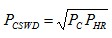## Ratio of Harmonic Means